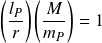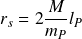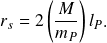The Schwarzschild radius defines a volume of space in which mass density is maximized. The elementary form of the equation is simply the mass density operator identity divided by 2. Setting the mass density operator

equal to one gives the identity.

Dividing the identity in half and re-arranging the formula gives the elementary form of the Schwarzschild radius formula.

The formula shows that the simple ratio between a given quantity of rest mass and the Planck mass is all that is needed to determine the region of space in which the mass density is maximized.

## Historical formula

The elementary formula is derived from the historical Schwarzschild radius formula which uses the gravitational constant. The historical form of the equationcan be re-stated in elementary form by replacing the gravitational constant with natural units of length, mass, and time. The elementary formulacan then be reduced to its simplest formThe following illustration shows how the historical formula transforms into the natural formula with operators and potential.

Gravitational force: conversion from historical to natural formula